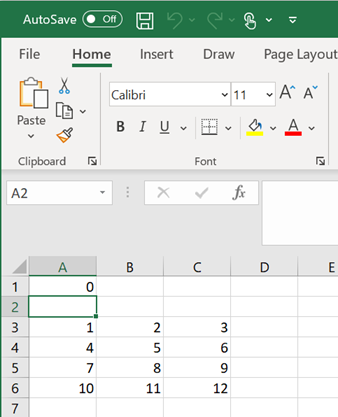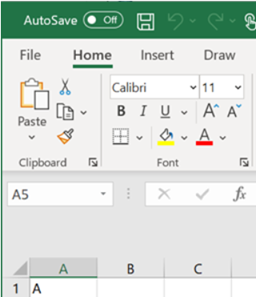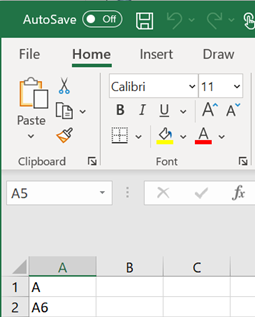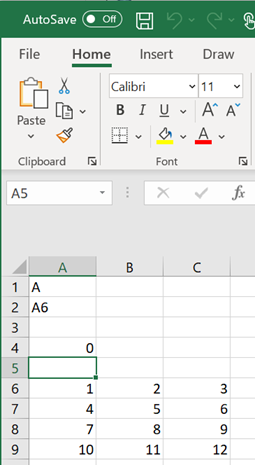# MLGetMatrix

Write contents of MATLAB matrix to Microsoft Excel worksheet

## Syntax

``= MLGetMatrix(var_name,edat)``
``MLGetMatrix var_name, edat``
``out = MLGetMatrix(var_name,edat)``

## Description

example

````= MLGetMatrix(var_name,edat)` writes the contents of MATLAB® matrix `var_name` in the Excel® worksheet, beginning in the upper-left cell specified by `edat`. Use this syntax when working directly in a worksheet.```

example

````MLGetMatrix var_name, edat` writes the contents of MATLAB matrix `var_name` in the Excel worksheet, beginning in the upper-left cell specified by `edat`. Use this syntax in a VBA macro.```
````out = MLGetMatrix(var_name,edat)` lets you find errors when you execute the `MLGetMatrix` function in a VBA macro. If the `MLGetMatrix` function fails, then `out` is a string containing an error code. Otherwise, `out` is `0`.```

## Examples

collapse all

Access the contents of a matrix in MATLAB and write the contents to a worksheet. Specify the name of the matrix and the destination cells explicitly.

Define a 4-by-3 matrix `A` in MATLAB.

```A = [1,2,3;4,5,6;7,8,9;10,11,12] ```
```A = 1 2 3 4 5 6 7 8 9 10 11 12 ```

Open Excel and make sure cell `A1` is selected in the worksheet. To write the contents of the MATLAB matrix `A` starting in cell `A3` of worksheet `Sheet1`, enter this text in cell `A1` and press Enter:

```= MLGetMatrix("A","Sheet1!A3") ```

The `MLGetMatrix` function fills the cells `A3` through `C6` with data from the matrix.Access the contents of a matrix in MATLAB and write the contents to a worksheet. Specify the destination for the matrix contents by using a cell reference.

Define a 4-by-3 matrix `A` in MATLAB.

```A = [1,2,3;4,5,6;7,8,9;10,11,12] ```
```A = 1 2 3 4 5 6 7 8 9 10 11 12 ```

Open Excel and enter the name of the matrix in cell `A1`.Enter the reference for the destination cell `A6` in cell `A2`.Make sure cell `A4` is selected. Access the MATLAB matrix named by the string in cell `A1` and write the contents of the matrix to the worksheet by using the reference in cell `A2`. Enter this text in cell `A4` and press Enter:

```= MLGetMatrix(A1,A2) ```

The `MLGetMatrix` function fills the cells `A6` through `C9` with data from the matrix.Access the contents of a matrix in MATLAB and write the contents to a worksheet using a VBA macro.

Define a 4-by-3 matrix `A` in MATLAB.

```A = [1,2,3;4,5,6;7,8,9;10,11,12] ```
```A = 1 2 3 4 5 6 7 8 9 10 11 12 ```

On the Developer tab in Excel, click in the Code group. The Visual Basic® Editor window opens.

From the Insert menu, select Module to insert a new module. In the Module1 window, enter this VBA code containing a macro named `GetMatrix`.

```Sub GetMatrix() MLGetMatrix "A","B2" MatlabRequest End Sub```

`MatlabRequest` initializes internal Spreadsheet Link™ variables and enables `MLGetMatrix` to work in a subroutine.

The `GetMatrix` macro uses the `MLGetMatrix` function to access the contents of matrix `A` from the MATLAB Workspace. Then, the function writes the contents of the matrix starting at cell `B2`. For details about working with modules, see Excel Help.

Run the macro by clicking the button on the VBA toolbar. For details about running macros, see Excel Help.

The `MLGetMatrix` function accesses the contents of the matrix and writes them to the worksheet.

## Input Arguments

collapse all

Name of the MATLAB matrix to access, specified as a string. `var_name` in quotes directly specifies the matrix name. `var_name` without quotes specifies a worksheet cell address (or range name) that contains the matrix name. Do not use the MATLAB variable `ans` as `var_name`.

Example: `"A"`

Example: `A2`

Location of the data in the worksheet where the function writes the contents of `var_name`, specified as a string.

`edat` in quotes directly specifies the location. `edat` without quotes specifies a worksheet cell address (or range name) that contains a reference to the location. In both cases, `edat` must be a cell address or a range name.

Example: `"B2"`

Example: `A6`

## Tips

• If data exists in the specified worksheet cells, it is overwritten.

• If the dimensions of the MATLAB matrix are larger than that of the specified cells, the data overflows into additional rows and columns.

• `edat` must not include the cell that contains the `MLGetMatrix` function. In other words, be careful not to overwrite the function itself. Also make sure the worksheet has enough room to write the matrix contents. If there is insufficient room, the function execution fails because the data has too many rows or columns. For details about the errors in the worksheet, see Worksheet Cell Errors.

• The `MLGetMatrix` function does not automatically adjust cell addresses. If `edat` is an explicit cell address, edit it to correct the address when you do either of the following:

• Insert or delete rows or columns.

• Move or copy the function to another cell.

• If worksheet calculation mode is automatic, `MLGetMatrix` executes when you enter the formula in a cell. If worksheet calculation mode is manual, enter the `MLGetMatrix` function in a cell, and then press to execute it. However, pressing in this situation may also execute other worksheet functions again and generate unpredictable results.

• If you use `MLGetMatrix` in a macro subroutine, enter `MatlabRequest` on the line after the `MLGetMatrix` command. `MatlabRequest` initializes internal Spreadsheet Link variables and enables `MLGetMatrix` to work in a subroutine. Do not include `MatlabRequest` in a macro function unless the function is called from a subroutine.

• To work with VBA code in Excel with Spreadsheet Link, you must enable Spreadsheet Link as a reference in the Microsoft® Visual Basic Editor. For details, see Installation.

## Version History

Introduced before R2006a